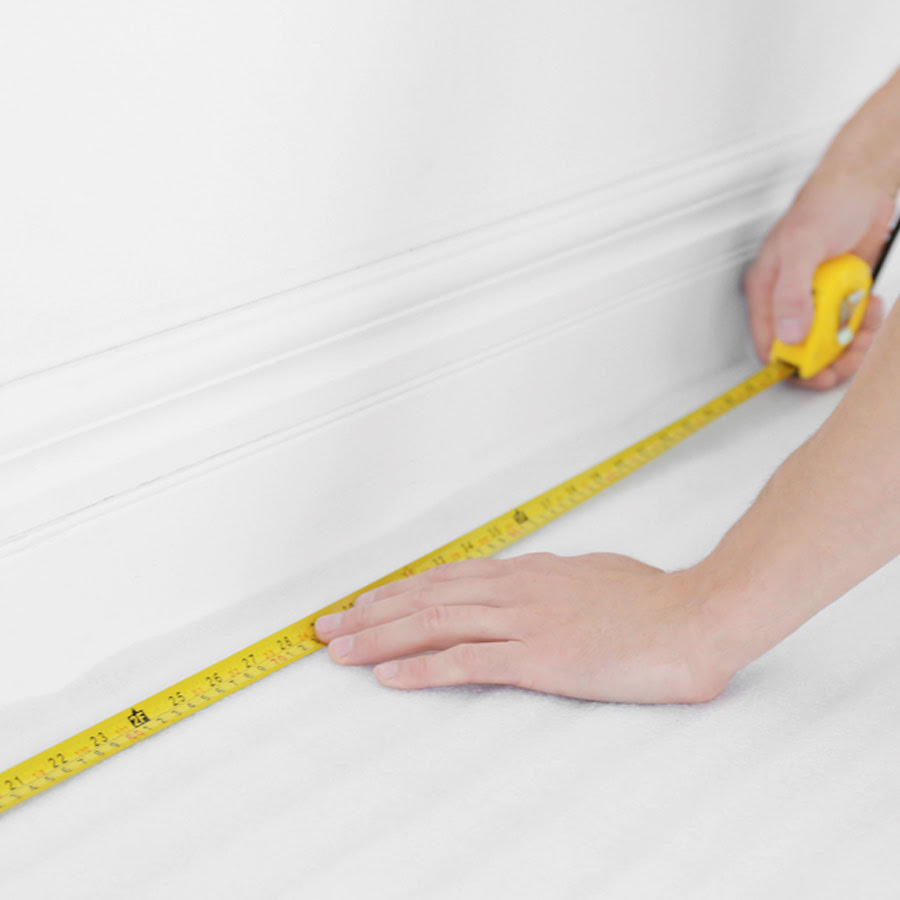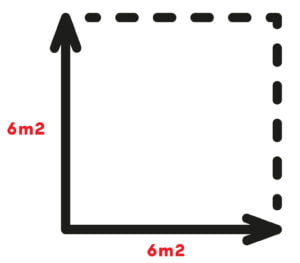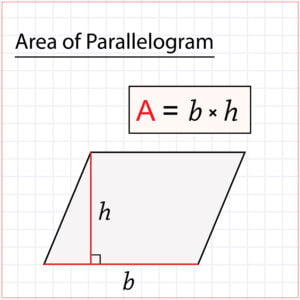# How to work out square metres for flooring – a super quick guideCalculating your room size is one of the first things to do when replacing your flooring. However, many rooms are different shapes and sizes as opposed to the simple square. Some might be  longer on one side than the other. Or it might even be split into different sections with each being, for example, a square shape.

## How to measure the size of a square or rectangular room

Square or rectangular rooms are the easiest of all rooms to measure.

Simply measure the width by the length and multiply the 2 together. For a rectangle, you would measure one of the shortest sides and one of the longest sides, then multiply those two together.

For example, the below room measures 6m x 6m. Meaning the total area is 36m2.Remember, if your room is this shape (and most hopefully are), then you can simply use our room calculator on any of our product pages to work out your room. Not only that, you can choose whether you want to do it in feet or metres before finding out the exact amount of underlay you’ll need

## Circular room

Round rooms are slightly more complicated. You need to bring in what’s called Pi or written as ‘π’

So, to work out the measurement for a circular room. You need to measure the width right across the centre of the room, then divide that number by 2

For example if the longest part of the room is 10m, then you would divide 10 by 2, which gives you 5m.

This is called the radius. Now that you have the radius, you need to multiply that by itself. In this example 5 x 5 = 25.

So the number you’re going to be working with for the next step is 25.

Now you need to multiply 25 by 3.142 (which is the value of Pi)

So to finalise the maths, it’s:

25 x 3.142 = 78.55 (80m2)

## sides not straight but parallel

If you have a room, which is shorter on one side than the other. Or maybe the sides of your room aren’t exactly parallel. Have no fear there’s an equation right here.

This type of shape in the mathematical world is known as a parallelogram. In this instance, you’ll want to calculate the base by the height. Note that means instead of calculating the side of the room, you’ll probably need to go up the middle of the room so the line is straight. Just as shown in the image below.You could always use this handy calculator to work out the room size as it will save you a bit of time.